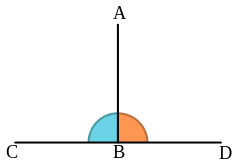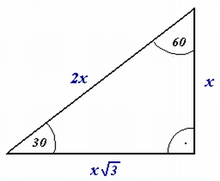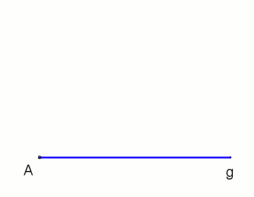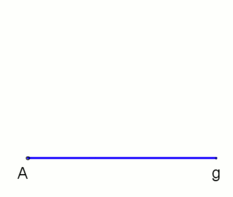# Right angle

For other uses, see Right angle (disambiguation).A right angle is equal to 90 degrees.A line segment (AB) drawn so that it forms right angles with a line (CD).

In geometry and trigonometry, a right angle is an angle that bisects the angle formed by two adjacent parts of a straight line. More precisely, if a ray is placed so that its endpoint is on a line and the adjacent angles are equal, then they are right angles. As a rotation, a right angle corresponds to a quarter turn (that is, a quarter of a full circle).

Closely related and important geometrical concepts are perpendicular lines, meaning lines that form right angles at their point of intersection, and orthogonality, which is the property of forming right angles, usually applied to vectors. The presence of a right angle in a triangle is the defining factor for right triangles, making the right angle basic to trigonometry.

The term is a calque of Latin angulus rectus; here rectus means "upright", referring to the vertical perpendicular to a horizontal base line.

## SymbolsRight triangle, with the right angle shown via a small square.Another option of diagrammatically indicating a right angle, using an angle curve and a small dot.

In Unicode, the symbol for a right angle is U+221F RIGHT ANGLE (HTML `&#8735;`). It should not be confused with the similarly shaped symbol U+231E BOTTOM LEFT CORNER (HTML `&#8990;`). Related symbols are U+22BE RIGHT ANGLE WITH ARC (HTML `&#8894;`), U+299C RIGHT ANGLE VARIANT WITH SQUARE (HTML `&#10652;`), and U+299D MEASURED RIGHT ANGLE WITH DOT (HTML `&#10653;`).

In diagrams, the fact that an angle is a right angle is usually expressed by adding a small right angle that forms a square with the angle in the diagram, as seen in the diagram of a right triangle (in British English, a right-angled triangle) to the right. The symbol for a measured angle, an arc, with a dot, is used in some European countries, including German-speaking countries and Poland, as an alternative symbol for a right angle.

## Euclid

Right angles are fundamental in Euclid's Elements. They are defined in Book 1, definition 10, which also defines perpendicular lines. Euclid uses right angles in definitions 11 and 12 to define acute angles (those smaller than a right angle) and obtuse angles (those greater than a right angle). Two angles are called complementary if their sum is a right angle.

Book 1 Postulate 4 states that all right angles are equal, which allows Euclid to use a right angle as a unit to measure other angles with. Euclid's commentator Proclus gave a proof of this postulate using the previous postulates, but it may be argued that this proof makes use of some hidden assumptions. Saccheri gave a proof as well but using a more explicit assumption. In Hilbert's axiomatization of geometry this statement is given as a theorem, but only after much groundwork. One may argue that, even if postulate 4 can be proven from the preceding ones, in the order that Euclid presents his material it is necessary to include it since without it postulate 5, which uses the right angle as a unit of measure, makes no sense.

## Conversion to other units

A right angle may be expressed in different units:

## Rule of 3-4-5

Throughout history carpenters and masons have known a quick way to confirm if an angle is a true "right angle." It is based on the most widely known Pythagorean triple (3, 4, 5) and so called the "Rule of 3-4-5." From the angle in question, running a straight line along one side exactly three units in length, and along the second side exactly four units in length, will create a hypotenuse (the longer line opposite the right angle that connects the two measured endpoints) of exactly 5 units in length. This measurement can be made quickly and without technical instruments. The geometric law behind the measurement is the Pythagorean theorem ("The square of the hypotenuse of a right triangle is equal to the sum of the squares of the two adjacent sides").

## Thales' theoremConstruction of the perpendicular to the straight line g at or through the point P (applicable not only at the end point A, M is freely selectable), animationAlternative construction if P outside of the straight line g and the distance A to P' is small (B is freely selectable), animation
Main article: Thales' theorem

Thales' theorem states that an angle inscribed in a semicircle (with a vertex on the semicircle and its defining rays going through the endpoints of the semicircle) is a right angle.

Two application examples in which the right angle and the Thales' theorem are included (see animations).Wikimedia Commons has media related to Right angles.